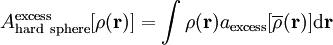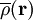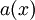Difference between revisions of "Tarazona's weighted density approximation"This article is a 'stub' page, it has no, or next to no, content. It is here at the moment to help form part of the structure of SklogWiki. If you add sufficient material to this article then please remove the {{Stub-general}} template from this page.$A_{\mathrm {hard~sphere}}^{\mathrm {excess}} [\rho ({\mathbf r})] = \int \rho ({\mathbf r}) a_{\mathrm {excess}} [\overline\rho ({\mathbf r})]{\mathrm d}{\mathbf r}$,
where$\overline\rho ({\mathbf r})$ is an average of the density distribution and where A is the Helmholtz energy function. The function$a(x)$ should vanish at low values of its argument (so that the excess vanishes and one is left with the ideal case), and diverge at some saturation density corresponding to complete packing.